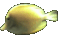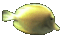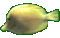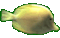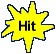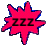Derivative Chain Rule for Composite Functions     Math Help Fun     Game Tips:

- A composite function such as y = (x3+x4)20 represents a function of a function.
In this case, y is a function of g. This means y = f(g) = g20 where g itself is a function of x ,  g = x3+x4.

- The derivative Chain Rule states that the derivative of y = f(g) is  dy/dx = (dy/dg)(dg/dx).
The chain rule, in words, says that the
derivative of y with respect to x=(derivative of y with respect to g)times(derivative of g with respect to x).
- Example 1:   y= (x3+x4)20 has the derivative dy/dx= (20g19)(3x2+4x3)
which equals (20(x3+x4)19)(3x2+4x3)

- Example 2:   the unsimplified derivative of y= (x5-2x)-7 is  dy/dx = (-7(x5-2x)-8)(5x4-2)

- Note: This game also uses the derivative Power rule for y=xn which gives the derivative  dy/dx = nxn-1.

- Your Game Score is reduced by the number of fish hits.

- To slow the game speed repeat tap/click on the word Slider.
- To increase the game speed repeat tap/click on the word Math.
- Speed can also be adjusted with a keyboard's - and + keys.

- Refresh/Reload the web page to restart the game.